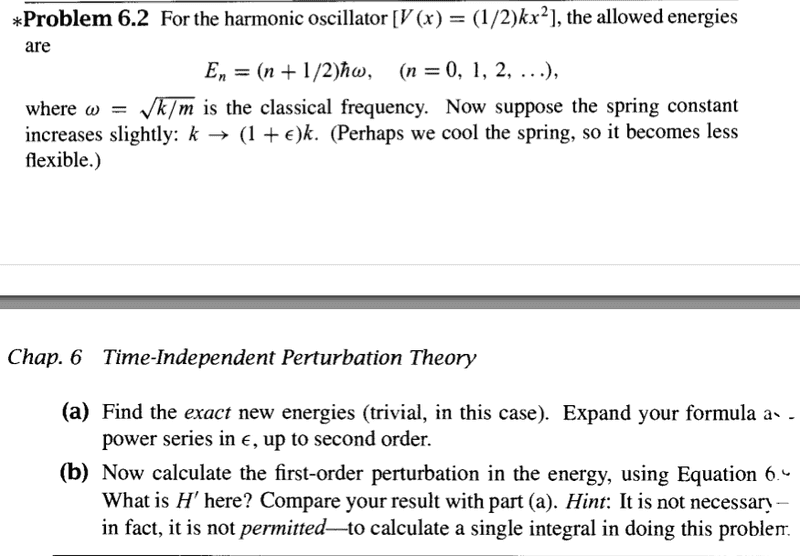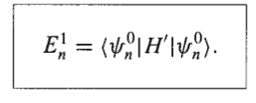# Perturbed in the harmonic oscillator

## Homework Statement## Homework Equations## The Attempt at a Solution

for part a I do not know how to write it in power series form ?

for part b :
I chose the perturbed H' is v(x)= (1+ε )K x^2 /2
then I started integrate E_1 = ∫ H' ψ^2 dx

the problem was , the result equals to ∞ !!

shall I integrate in the interval [ 0,∞ ] !

Last edited by a moderator:

## Answers and Replies

pasmith
Homework Helper
for part a I do not know how to write it in power series form ?

You are given $E_n = (n + \frac12)\hbar\sqrt{k/m}$. $k$ is now $k(1+\epsilon)$, so $E_n$ is now
$$E_n = \left(n + \frac12\right)\hbar\sqrt{\frac km(1 + \epsilon)} = \left(n + \frac12\right)\hbar\sqrt{\frac km}(1 + \epsilon)^{1/2}$$
Since $|\epsilon| < 1$, $(1 + \epsilon)^{1/2}$ can be expanded in a binomial series.

ok , I do it thanks

any help for second part please

pasmith
Homework Helper
ok , I do it thanks

any help for second part please

I can't help you with that unless you tell me how your textbook defines $H'$, $E_n^1$ and $\psi_n^0$. I am prepared to guess that you have
$$E_n = E_n^0 + \epsilon E_n^1 + O(\epsilon^2)\\ \psi_n = \psi_n^0 +\epsilon\psi_n^1 + O(\epsilon^2)$$
but I'm not prepared to guess what $H'$ might be. However I suspect the method is to substitute the above into the Schrodinger equation to get
$$(E_n^0 + \epsilon E_n^1)(\psi_n^0 + \epsilon \psi_n^1) = -\frac{\hbar^2}{2m}\frac{\mathrm{d}^2}{\mathrm{d}x^2} (\psi_n^0 + \epsilon\psi_n^1) + \frac{kx^2}{2}(1 + \epsilon)(\psi_n^0 +\epsilon\psi_n^1)$$
and then require that the coefficients of $\epsilon^0$ and $\epsilon^1$ should vanish. At some stage you may want to take an inner product with $\langle\psi_n^0|$, and recall that
$$H_0 = -\frac{\hbar^2}{2m}\frac{\mathrm{d}^2}{\mathrm{d}x^2} + \frac{kx^2}{2}$$
is self-adjoint ($\langle f | H_0 | g \rangle = \langle g | H_0 | f \rangle$ for all $f$ and $g$).

vela
Staff Emeritus
Science Advisor
Homework Helper
Education Advisor
Your H' is wrong, or you're not expressing yourself clearly. H' is the difference between the complete Hamiltonian and the unperturbed Hamiltonian.

Show us how you're getting that the integral diverges. The problem seems to lie in your evaluation of the integral.